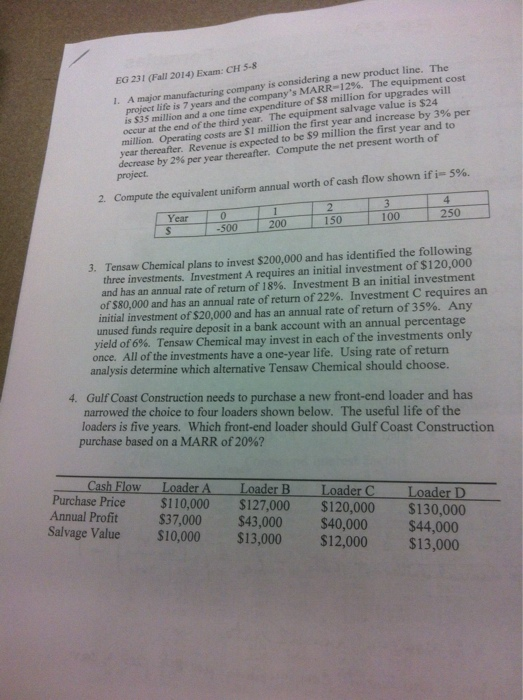# Tensaw Chemical plans Investment Decision Analysis

\$1.00Text: Tensaw Chemical plans to invest \$200,000 and has identified the following three investments. Investment A requires an initial investment of \$ 120,000 and has an annual rate of return of 18%. Investment B an initial investment of \$80,000 and has an annual rate of return of 22%. Investment C requires an initial investment of \$20,000 and has an annual rate of return of 35%. Any unused funds require deposit in a bank account with an annual percentage yield of 6%. Tensaw Chemical may invest in each of the investments only once. All of the investments have a one-year life.

Using rate of return analysis determine which alternative Tensaw Chemical should choose.

SKU: tensaw-chemical-plans-investment-decision-analysis Category: